# High School Physics : Understand Power

## Example Questions

### Example Question #2 : Understanding Power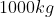sports car accelerates from rest to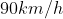in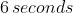.  What is the average power delivered by the engine?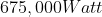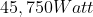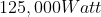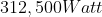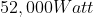Explanation:

Power is equal to the work done divided by how much time to complete that work.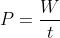Work is equal to the change in kinetic energy of an object.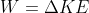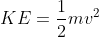We can solve for the work by solving for the change in kinetic energy.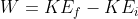Since the initial velocity is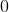, this can be dropped from the equation.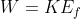needs to be converted to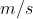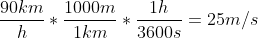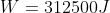We can now plug this into the power equation.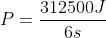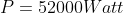The average power is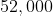Watts.

### Example Question #1 : Understand Power

Of the following, which is not a unit of power?

Watt

newton meter /second

joule/second

watt/second

watt/second

Explanation:

The unit for power is the Watt.  A watt is a measure of the Joules per second that an object uses.  Joules can also be written as Newton Meters.  Therefore a watt could also be considered a Newton Meter/Second.  The incorrect answer is the watt/second since watt is the base unit of power on its own.

### Example Question #5 : Understanding Power

To accelerate your car at a constant acceleration, the car’s engine must

Maintain a constant power output

Develop ever decreasing power

Maintain a constant turning speed

Develop ever increasing power

Maintain a constant power output

Explanation:

Power is equal to the Work put into the system per unit time.

Work is equal to the force acting on the object multiplied by the displacement through which it acts.

Therefore power is directly related to the force applied.

Force is also directly related to the acceleration of an object.   A constant force will create a constant acceleration.

Since power is directly related to the force applied, and the force must be constant to maintain a constant acceleration, the power must also therefore be constant.

### Example Question #2 : Power

A bicyclist coasts down a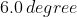hill at a steady speed of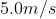.  Assuming a total mass of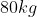(bike plus rider) what must be the cyclists’s power output to climb the same hill at the same speed?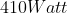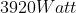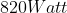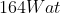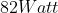Explanation:

First we need to analyze the motion of the rider on the way down the incline.  During this time, the bicyclist has a constant velocity, which means that all forces acting on him must be balanced.  He has a portion of the force of gravity pulling him down the hill and the force of friction opposing his motion.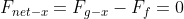We can use components to find the force of gravity in the x direction.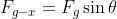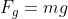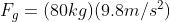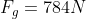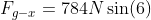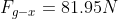Since the net force is equal towe know that the force of gravity in the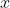direction is equal to the force of friction.

Now let us consider how the bicyclist will travel when he goes up the hill.  This time, the forces acting on the bicyclist are the force of gravity and friction resisting his motion and his applied force going up the hill.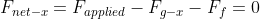The force of friction and the force of gravity are the same as when the cyclist was coasting down the hill.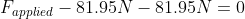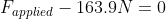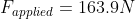The bicyclist doesn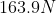of work to get back up the hill.  We can now use this in our power equation to determine the amount of power used.

Power is equal to the work divided by the time to complete the work.Work is equal to the force times the displacement through which the object moved.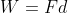We can substitute this into our power equation to get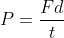Velocity is equal to the distance over the time.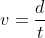Therefore power could be written as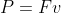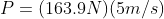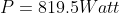### Example Question #3 : Power

How long will it take an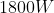motor to lift a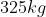piano to a window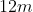above?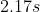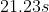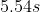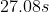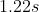Explanation:

Power is equal to the work divided by the time it takes to complete the work.Work is equal to the force times the displacement.In this case the motor is lifting the piano.  Therefore the force that it is exerting is equal to the force of gravity pulling the piano down.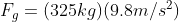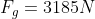We can now calculate the work done on the piano.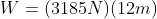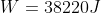We can now plug this value into the power equation and solve for the time.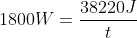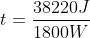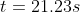It will take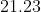seconds to the lift the piano.

### Example Question #4 : Power

The quantity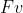is

The kinetic energy of the object

The work done on the object by the force

The potential energy of the object

The power supplied to object by the force

The power supplied to object by the force

Explanation:

Power is equal to the work divided by the time to complete the work.Work is equal to the force times the displacement through which the object moved.We can substitute this into our power equation to getVelocity is equal to the distance over the time.Therefore power could be written as### All High School Physics Resources